# Nichols Plot

Constant magnitude loci that are M-circles and constant phase angle loci that are N-circles are the fundamental components in designing the Nichols chart. The constant M and constant N circles in G (jω) plane can be used for the analysis and design of control systems. However the constant M and constant N circles in gain phase plane are prepared for system design and analysis as these plots supply information with fewer manipulations. Gain phase plane is the graph having gain in decibel along the ordinate (vertical axis) and phase angle along the abscissa (horizontal axis). The M and N circles of G (jω) in the gain phase plane are transformed into M and N contours in rectangular co-ordinates. A point on the constant M loci in G (jω) plane is transferred to gain phase plane by drawing the vector directed from the origin of G (jω) plane to a particular point on M circle and then measuring the length in db and angle in degree.
The critical point in G (jω), plane corresponds to the point of zero decibel and -180o in the gain phase plane. Plot of M and N circles in gain phase plane is known as Nichols chart /plot.

The Nichols plot is named after the American engineer N.B Nichols who formulated this plot. Compensators can be designed using Nichols plot. Nichols plot technique is however also used in designing of dc motor. This is used in signal processing and control design. Nyquist plot in complex plane shows how phase of transfer function and frequency variation of magnitude are related. We can find out the gain and phase for a given frequency. Angle of positive real axis determines the phase and distance from origin of complex plane determines the gain. There are some advantages of Nichols plot in control system engineering. They are:
Gain and phase margin can be determined easily and also graphically.
Closed loop frequency response is obtained from open loop frequency response.
Gain of the system can be adjusted to suitable values.
Nichols chart provides frequency domain specifications.

There are some drawbacks of Nichols plot also. Using Nichols plot small changes in gain cannot be encountered easily.
Constant M and N circles in the Nichols chart are deformed into squashed circles. The complete Nichols chart extends for the phase angle of G (jω) from 0 to -360o. The region of ∠G(jω) used for analysis of systems in between -90o to -270o. These curves repeat after every 180o interval.
If the open loop T.F of unity feedback system G(s) is expressed as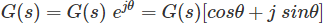Closed loop T.F isSubstituting s = jω in the above eq. frequency functions are,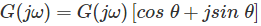and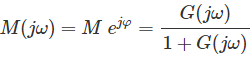Eliminating G(jω) from above two eq.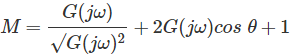and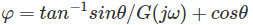Want To Learn Faster? 🎓
Get electrical articles delivered to your inbox every week.
No credit card required—it’s 100% free.